# Xbar S Chart with JMP

### What is a Xbar S Chart?

The X-S chart (also called Xbar S chart) is a control chart for continuous data with a constant subgroup size greater than ten. The Xbar chart plots the average of a subgroup as a data point. The S chart plots the standard deviation within a subgroup as a data point.
The Xbar chart monitors the process mean and the S chart monitors the variability within subgroups. The Xbar is valid only if the S chart is in control. The underlying distribution of the Xbar-S chart is normal distribution.

### Xbar Chart Equations

Xbar Chart

Data Point: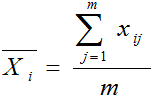Center Line: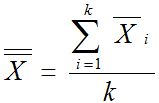Control Limits: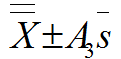Where:

• m is the subgroup size
• k is the number of subgroups
• A3 is a constant depending on the subgroup size

### S Chart Equations

S chart

Data Point: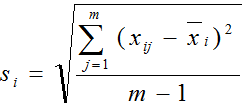Center Line: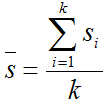Upper Control Limit: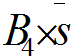Lower Control Limit: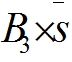Where:

• m is the subgroup size
• k is the number of subgroups
• B3 and B4 are constants depending on the subgroup size

### Use JMP to Plot Xbar-S Charts

Data File: “XbarS.jmp”

#### Steps to plot Xbar-S charts in JMP:

1. Analyze -> Quality & Process -> Control Chart -> XBar
2. Select Measurement in the Process Field
3. Select Subgroup ID in the Sample Label Field
4. Be sure the check the Xbar and S check boxes
5. Click Ok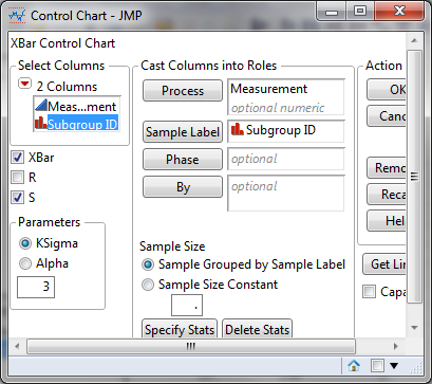### Xbar-S Charts Diagnosis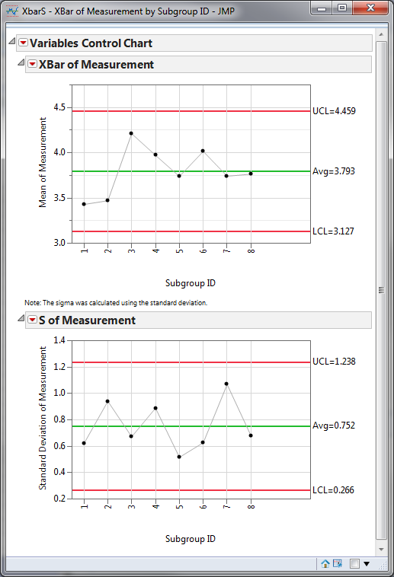Model summary: Since the S chart is in control, the Xbar chart is valid. In b

oth charts, there are not any data points failing any tests for special causes (i.e. all the data points fall between the control limits and spread around the center line with a random pattern). We conclude that the proces

s is in control.